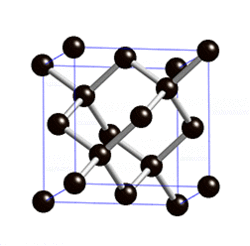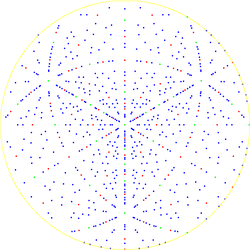# Diamond cubic

Diamond cubicUnit cell of the diamond cubic crystal structurePole figure in stereographic projection of the diamond lattice showing the 3-fold symmetry along the  direction.

The diamond cubic crystal structure is a repeating pattern of 8 atoms that certain materials may adopt as they solidify. While the first known example was diamond, other elements in group IV also adopt this structure, including tin, the semiconductors silicon and germanium, and silicon/germanium alloys in any proportion.

## Crystallographic structure

Diamond cubic is in the Fd3m space group, which follows the face-centered cubic bravais lattice. The lattice describes the repeat pattern; for diamond cubic crystals this lattice is "decorated" with a motif of two tetrahedrally bonded atoms in each primitive cell, separated by 1/4 of the width of the unit cell in each dimension. Many compound semiconductors such as gallium arsenide, β-silicon carbide and indium antimonide adopt the analogous zincblende structure, where each atom has nearest neighbors of an unlike element. Zincblende's space group is F43m, but many of its structural properties are quite similar to the diamond structure.

The atomic packing factor of the diamond cubic structure (the proportion of space that would be filled by spheres that are centered on the vertices of the structure and are as large as possible without overlapping) is$\frac{\pi\sqrt{3}}{16} \approx 0.34$, significantly smaller (indicating a less dense structure) than the packing factors for the face-centered and body-centered cubic lattices. Zinc blende structures have higher packing factors than 0.34 depending on the relative sizes of their two component atoms.

The first, second, third and fourth nearest-neighbor distances in units of the cubic lattice constant are$\frac{\sqrt{3}}{4}$,$\frac{\sqrt{2}}{2}$,$\frac{\sqrt{11}}{4}$, and 1, respectively.

## Mathematical structure

Mathematically, the points of the diamond cubic structure can be given coordinates as a subset of a three-dimensional integer lattice by using a cubical unit cell four units across. With these coordinates, the points of the structure have coordinates (xyz) satisfying the equations

x = y = z (mod 2), and
x + y + z = 0 or 1 (mod 4).

There are eight points (modulo 4) that satisfy these conditions:

(0,0,0), (0,2,2), (2,0,2), (2,2,0),
(3,3,3), (3,1,1), (1,3,1), (1,1,3)

All of the other points in the structure may be obtained by adding multiples of four to the x, y, and z coordinates of these eight points. Adjacent points in this structure are at distance √3 apart in the integer lattice; the edges of the diamond structure lie along the body diagonals of the integer grid cubes. This structure may be scaled to a cubical unit cell that is some number a of units across by multiplying all coordinates by a/4.

Alternatively, each point of the diamond cubic structure may be given four-dimensional integer coordinates that sum to either zero or one. Two points are adjacent in the diamond structure if and only if their four-dimensional coordinates differ by one in a single coordinate. The total difference in coordinate values between any two points (their four-dimensional Manhattan distance) gives the number of edges in the shortest path between them in the diamond structure. The four nearest neighbors of each point may be obtained, in this coordinate system, by adding one to each of the four coordinates, or by subtracting one from each of the four coordinates, accordingly as the coordinate sum is zero or one. These four-dimensional coordinates may be transformed into three-dimensional coordinates by the formula

(a, b, c, d) → (a + bcd, ab + cd, −a + b + cd).

Because the diamond structure forms a distance-preserving subset of the four-dimensional integer lattice, it is a partial cube.

Yet another coordinatization of the diamond cubic involves the removal of some of the edges from a three-dimensional grid graph. In this coordinatization, which has a distorted geometry from the standard diamond cubic structure but has the same topological structure, the vertices of the diamond cubic are represented by all possible 3d grid points and the edges of the diamond cubic are represented by a subset of the 3d grid edges.

The diamond cubic is sometimes called the "diamond lattice" but it is not, mathematically, a lattice: there is no translational symmetry that takes the point (0,0,0) into the point (3,3,3), for instance. However, it is still a highly symmetric structure: any incident pair of a vertex and edge can be transformed into any other incident pair by a congruence of Euclidean space.

## Manufacturing considerationsA diamond cubic crystal viewed from a <110> direction.

Since materials with diamond or zinc blende structures are important for formation of wafers used in the fabrication of modern electronics, it is important to know that they present open, hexagonal ion channels when ion implantation is carried out from any of the <110> directions (that is, 45° from one of the cube edges). Their open structure also results in a volume reduction upon melting or amorphization, as is also seen in ice.

They display octahedral cleavage, which means that they have four planes—directions following the faces of the octahedron where there are fewer bonds and therefore points of structural weakness—along which single crystals can easily split, leaving smooth surfaces. Similarly, this lack of bonds can guide chemical etching of the right chemistry (i.e., potassium hydroxide solutions for Si) to produce pyramidal structures such as mesas, points, or etch pits, a useful technique for microelectromechanical systems.

Wikimedia Foundation. 2010.

### Look at other dictionaries:

• Diamond (disambiguation) — Diamond is the hardest known natural material. Diamond, ♦ or ◊ may also refer to: Diamond (gemstone) Diamonds, a suit of cards Alt codes, ♦, the character or symbol formed by using the Alt key in conjunction with the Number Pad (Alt+num4) Lozenge …   Wikipedia

• Cubic zirconia — A round brilliant cut cubic zirconia Cubic zirconia (or CZ) is the cubic crystalline form of zirconium dioxide (ZrO2). The synthesized material is hard, optically flawless and usually colorless, but may be made in a variety of different colors.… …   Wikipedia

• Diamond simulant — Due to its low cost and close visual likeness to diamond, cubic zirconia has remained the most gemologically and economically important diamond simulant since 1976. The high price of gem grade diamonds, as well as significant ethical concerns of… …   Wikipedia

• Cubic crystal system — A rock containing three crystals of pyrite (FeS2). The crystal structure of pyrite is simple cubic, and this is reflected in the cubic symmetry of its natural crystal facets …   Wikipedia

• Diamond flaws — Contents 1 External flaws 1.1 Blemishes 1.2 Scratches 1.3 …   Wikipedia

• Diamond Nexus Labs — is a producer and marketer of laboratory produced gemstones. The company owns the trademark: “The World Leader in Laboratory Created Precious Gemstones”. The company produces and sells laboratory created rubies, emeralds sapphires and canary… …   Wikipedia

• Diamond Peak (Oregon) — Diamond Peak Diamond Peak Volcano, with the smaller Mount Yoran to the right Elevation 8,744 ft (2,665 m)  …   Wikipedia

• Diamond-like carbon — A ta C thin film on silicon (15 mm diameter) exhibiting regions of 40 nm and 80 nm thickness …   Wikipedia

• Diamond Valley Lake — Location Riverside …   Wikipedia